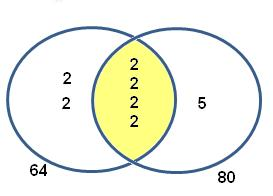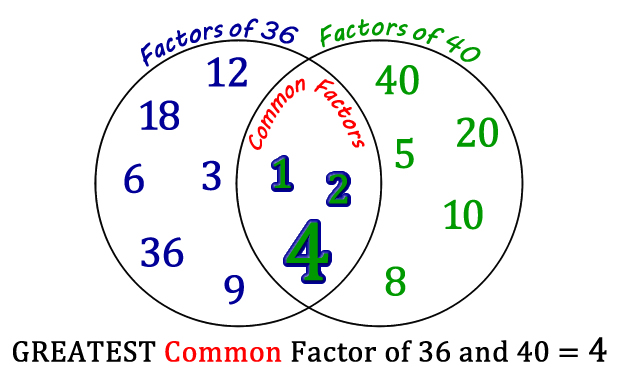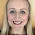## 21 March 2015

### Tricks and Tips 1: HCF

I recently presented a workshop at the National Mathematics Teacher Conference (#mathsconf2015) entitled 'Tricks and Tips: Clever Methods for Explaining Mathematical Concepts'. This post summarises the content of that workshop for those who were unable to attend. I have quite a lot to cover so I expect I'll need to write three or four blog posts. In this one I'm going to explain the rationale for the workshop and describe alternative methods for finding a Highest Common Factor. In subsequent posts I'll cover sequences, linear graphs, surds, quadratics, compound measures and a few more bits and pieces.
Workshop aim
How do you find the Highest Common Factor of two numbers? Do you use a Venn method? List the factors? Use the Euclidean Algorithm? Are there 'better' methods that you don't know about? (how do we define 'better'?). These are the sort of questions I want to explore. The aim of my workshop was to encourage people to reflect on their subject knowledge and the effectiveness of their explanations. I also hoped that everyone would learn new methods that they might consider using at school.

What determines the way we choose the explain things?
Most teachers establish teaching habits during their training and NQT year. The method they use the first time they teach a topic will probably stay with them throughout their career - unless they make a concious effort to try a new method.

One of the few things I remember about GCSE maths was that I solved equations by 'moving terms over the equals sign' (the 'magic portal method'). This method is now considered to be a shortcut which stands in the way of conceptual understanding. These days it is more acceptable to teach students to solve equations using inverse operations (ie balance the equation by 'doing the same thing' to both sides). It's lucky that during my PGCE someone told me not to use the magic portal method because before then I was convinced that it was 'the' way to solve equations.Source: https://mrjasonto.wordpress.com
When we were student teachers, we drew our methods from a variety of sources. ITT courses don't cover much in the way of mathematical methods, so we were left to gather ideas from examples in textbooks, our memories from school, observations of teachers and conversations with colleagues. It's these conversations with colleagues that are vital, but we simply don't get enough time for them. Most of the new methods I've encountered over the years have not been through organised CPD (ie 'collaboration sessions') within my department, but instead through chance encounters. My first ever blog post was about an alternative method for matrix multiplication that I'd happened to stumble across online.
During my PGCE I was asked to teach a lesson on Highest Common Factor. I remember my mentor showing me the 'Venn Method'. As a result of that conversation I used the Venn Method for years, until by chance a colleague mentioned an alternative. Let's look at that alternative method now, and a number of others. Will you stick with the method you know and love, or will you try something new?
Highest Common Factor and Lowest Common Multiple
I've identified six methods for finding the HCF and LCM of two numbers. I'll explain each method here and identify any pros and cons.

1. ListingSource: mathx.net
There's no harm in the listing method. It's brilliant in terms of underlying conceptual understanding - students can see exactly what they're trying to achieve here. List all the factors of the two numbers and find the biggest number that's in both lists - that's your HCF. List all the multiples of two numbers and find the smallest number that's in both lists - that's your LCM. Simple! Shame it's so time-consuming. And, in my experience, students sometimes miss factors from their list. One way to avoid this is by listing factors using a pairing method like this:
Factor rainbows are a pretty alternative (see this article from the NCTM).
2. The Venn Method
This is a popular method in the UK. First, we need to do a prime factor breakdown. By the way, if you're teaching prime factorisation then you might like these lovely factor tree activities from Don Steward.

Once you have the prime factors of each number, draw a Venn diagram and place the common factors in the intersection of two sets, as shown in the example below.tutorvista.com
The HCF is the product of the elements in A intersection B (ie 2 x 2 x 2 x 2 in the example above) and the LCM is the the product of all the elements in A union B (ie 2 x 2 x 2 x 2 x 2 x 2 x 5). Note that UK GCSE students are not yet familiar with this terminology (ie intersection and union), but they will be under the new GCSE syllabus.

Even though I taught the Venn method for years, I'm not a huge fan of it. In my experience, students are ok with filling in the Venn diagram but then they often can't remember which 'bit' is the HCF. If they do remember the method then they probably don't have a clue why it works.

Confusingly, it seems that some people use a different Venn method which involves putting all factors (not prime factors) into a Venn diagram and identifying the highest factor in the intersection (see example below). This is another form of the listing method described above - it's just a different way of organising the list. Let's call this Lenn Method - it's a hybrid of Venn and Listing.http://edtech2.boisestate.edu/brianroska/506/finalproject/gcf.html
3. Prime Factor Pairing
An alternative to the Venn Method is to do the prime factorisation but then skip the Venn. Write the prime factors of each number out as shown in the example below so it's easy to see which factors appear in both number - the product of these is the HCF. This method is featured in this post by Don Steward.
4. Euclidean Algorithm
I love the subtraction-based Euclidean Algorithm. It sounds complicated but it's incredibly easy. Try a few examples yourself to see how straightforward it is.
The method (including why it works) is explained in James Tanton's video below. I really like this method but for some reason I'm hesitant to use it with students... Would you?

Note that this method doesn't give you the Lowest Common Multiple, but it's easily found once you've got the Highest Common Factor.
This looks like a pain, but cancelling helps - in the example above I found the HCF of 60 and 84, so to find the LCM I multiply 60 by 84 and divide by the HCF.
5. The Indian Method
I've stopped using the Venn Method at school and now use this instead. I'm not sure it's really called the Indian Method, I'm only calling it that because of this video. At my school we call it the Korean Method because a Korean student introduced it to us! I've found that my students really like it. It's hard to go wrong. It's easy to explore why it works too.
Say we want to find the Highest Common Factor and Lowest Common Multiple of 315 and 420. Write down the two numbers, then (to the left, as in my example above) write down any common factor. I've chosen 5. Now divide 315 and 420 by 5 and write the answers underneath (63 and 84 in this case). Keep repeating this process until the two numbers have no common factors (ie 3 and 4 above). Now, your Highest Common Factor is simply the product of numbers on the left. And for the Lowest Common Multiple, find the product of the numbers on the left and the numbers in the bottom row (to find the LCM, look for the L shape).

6. The 'Upside Down Birthday Cake Method'
I should mention this method because I keep spotting it online (video here). The only difference between this and the Indian Method is that here we can only remove prime factors. This seems unnecessary - the Indian Method is quicker. In the example below, why divide by 2 if you spot larger common factors? Why not start by dividing by 4, 6 or 12?Source: https://uk.pinterest.com/pin/13229392628819982/
So that's it - six methods for finding a HCF and LCM.

Integers
I really like this set of questions from Don Steward. The following question confused one of my brightest Year 10s:

"A person has a rectangular plot of land measuring 8.4m by 5.6m. To survey the number of dandelions they want to divide it equally into the minimum number of square plots. What is the size of each square plot and how many such squares will there be?"

My student's approach to this question was to attempt to find the HCF of 8.4 and 5.6 using the Indian Method. This is what she did:
But she realised that her answer made no sense. 28 can't be the HCF of 8.4 and 5.6. Can you see where she went wrong? Although you can divide 8.4 by 7 (indeed, you can divide 8.4 by anything), it doesn't mean than 7 is a factor of 8.4. The numbers on the left must be factors of the numbers at the top. Non-integers don't have factors. A better approach would have been to convert the measurements to centimetres as shown below.
Factors vs Multiples
If your students struggle to remember the difference between factors and multiples then they might find this helpful: for factors, think of a factory (where separate parts are put together). For multiples, think of multi-packs eg if cokes are sold in multi-packs of 6 then I can buy 6, 12, 18 etc. These ideas are taken from this resource from the thechalkface.net.

ConclusionDid you know all of these methods? Will you try something new? Please let me know of any good methods that I've missed.

Even if you decide to stick with the method you're currently using, at least you've now reflected on how you teach this topic. Teachers rarely have the opportunity to pause and reflect.

This post should be read alongside Ed Southall's post Complements #9 LCM and HCF which explains the underlying concepts.

The whole presentation from my workshop is here. In my next post (give me a few days to write it!) I'll cover methods for teaching sequences, linear graphs and surds.

1.I do it a different way. It is similar to prime pairing but not the same.
Write each number as the product of its prime factors in index form.
Put a ring around the highest power of each prime. Multiply the ringed numbers to get the LCM and multiply the non-ringed numbers to get the HCF.
eg
24 = [2^3] x 3
36 = 2^2 x [3^2]

LCM = 2^3 x 3^2 = 72
HCF = 2^2 x 3 = 12

1.Yes a name for it is Winners and Losers - Winners LCM and Losers HCF

2.Response to my post from Dave Gale (@reflectivemaths):

https://reflectivemaths.wordpress.com/2015/03/21/hcf-and-lcm-resourceaholic/

3.Wow! I love finding 'new' ways and trying them out! I'm going to show the Indian method to my department tomorrow and see what they think. More importantly I'm going to try it with my GCSEs retake class who really benefit from different approaches that they potentially work better for them. Thank you for sharing

1.4.This is an excellent post. I used to use a listing method until I was shown the Venn method and have been using that ever since. I was looking at my son's book and noticed his teacher uses the Indian method instead of a factor tree to do prime factor decomposition of a single number. I had no idea it could be extended in this way to two numbers and love that you can short cut by taking out any common factor, not just primes. Thank you for sharing.

1.5.great post

6.5. The Indian Method is a nice method to teach BUT it appears to fall down in calculating the LCM of 3 numbers.
Is finding the LCM of 3 numbers in the new GCSE syllabus?

1.16|64 70 48
4|4 35 3
35|1 35 3
|1 1 3
3|1 1 1
LCM =16*4*35*3=6720

7.The Indian Method does work for more than two numbers, however, you need to use two slightly different approaches to find the HCF and LCM. For HCF, find the HCF of two of the numbers and then the HCF of that number and the third. For LCM you use the "normal" Indian method but you have to continue dividing until you have removed any duplicates or composite number from the horizontal. (See https://www.youtube.com/watch?v=z5vlj06Ex0U). This somewhat negates the benefit of the method over others, to my mind. Although it is certainly still and interesting one!

1.Thanks for clearing that up!

8.Thanks for helping me with LCM & HCF.You cleared a demon that had settled in my since I was 8 years old

9.Amazing material

10.When using the upside down cake method, you can use any common factor. You don't have to use prime factors. I've been teaching it this way ever since I started teaching. Students like it. You can also use it to rewrite a sum as a product of the GCF and the sum of 2 other numbers with no common factor. For example, I tell students to put the sum inside the cake and continue with the method. Once they complete the process, the numbers on the side of the cake would be multiplied to obtain the GCF and the bottom cake layer is the sum that would go in parenthesis beside the GCF. For example, 36 + 48 would go into the cake. Continue the process and the bottom layer would have 6 + 7 and the GCF would be 6. So the final answer would be written as 6(6 + 7).

11.Thank you for this article. I love showing the prime factor decomposition method as it seems to get to the heart of what each number is composed of but I find it an inefficient method for students to use. So for practical use I also like to teach the factoring method (Indian Method). What do you think to using calculator techniques such as the one here: https://youtu.be/mwpjSn-2gJM ?

1.That’s really interesting, thanks for sharing. By coincidence I’m just writing another hcf post so this is useful! I like the link to fractions.